• Slides: 15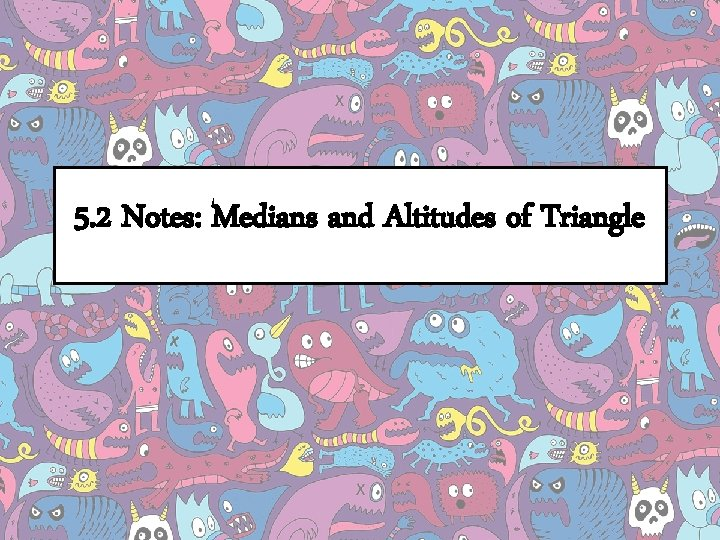5. 2 Notes: Medians and Altitudes of TriangleThe length of this snickers bar is 18 cm. What is 2/3 the length? 18 cm. What is 1/3 of the length of the snickers bar?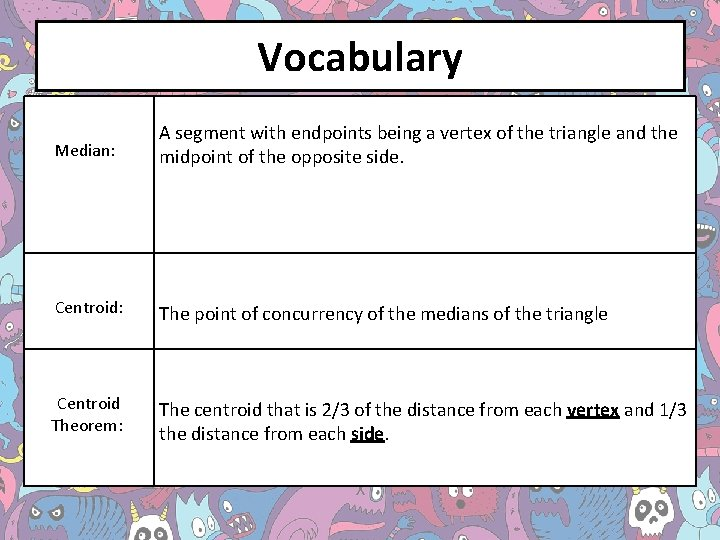Vocabulary Median: A segment with endpoints being a vertex of the triangle and the midpoint of the opposite side. Centroid: The point of concurrency of the medians of the triangle Centroid Theorem: The centroid that is 2/3 of the distance from each vertex and 1/3 the distance from each side.Example 1: In ΔXYZ, P is the centroid and YV = 12. Find YP and PV. Given the information about triangle XYZ, how can I mark up the triangle if knowing P is the centroid?Example 2: In ΔABC, CG = 4. Find GE. Given the picture of the triangle, what is G in my picture?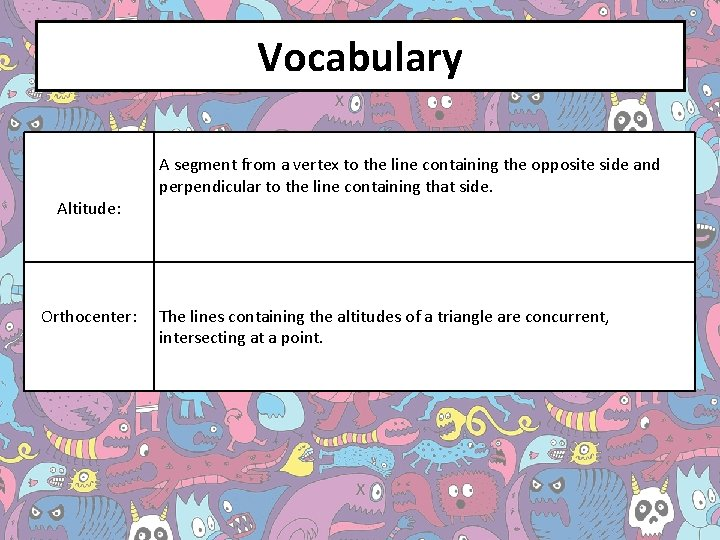Vocabulary Altitude: Orthocenter: A segment from a vertex to the line containing the opposite side and perpendicular to the line containing that side. The lines containing the altitudes of a triangle are concurrent, intersecting at a point.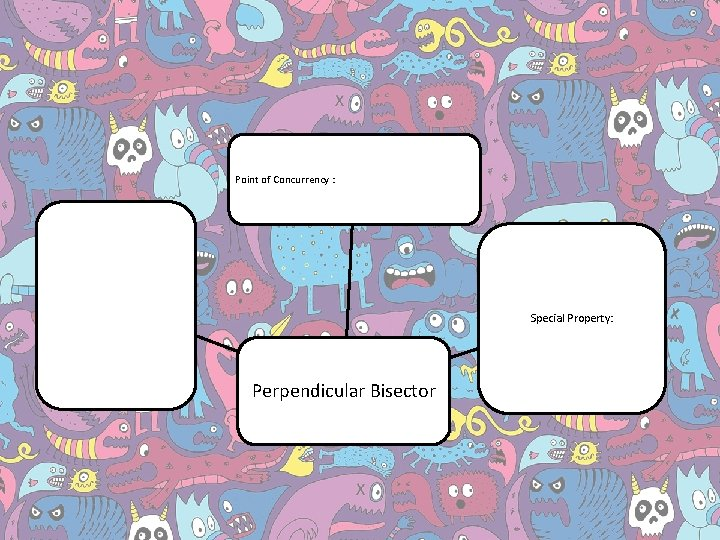Point of Concurrency : Special Property: Perpendicular Bisector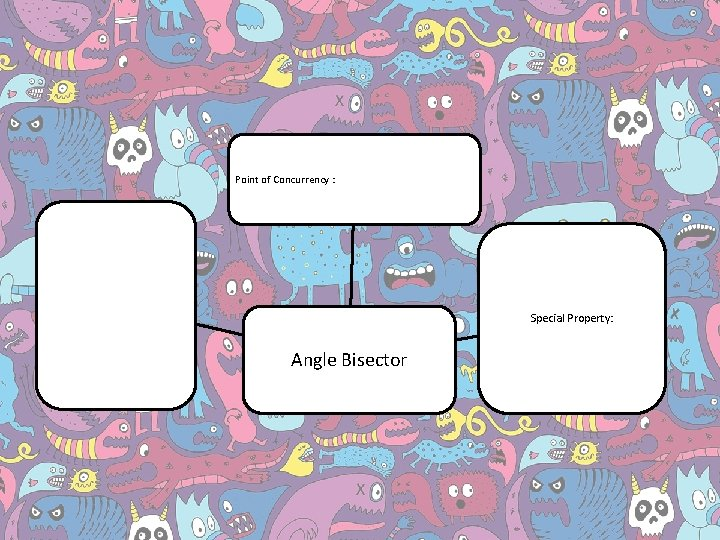Point of Concurrency : Special Property: Angle Bisector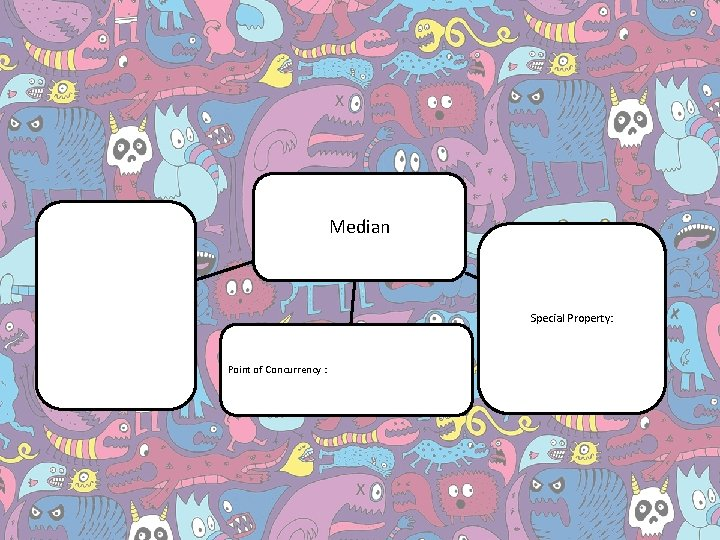Median Special Property: Point of Concurrency :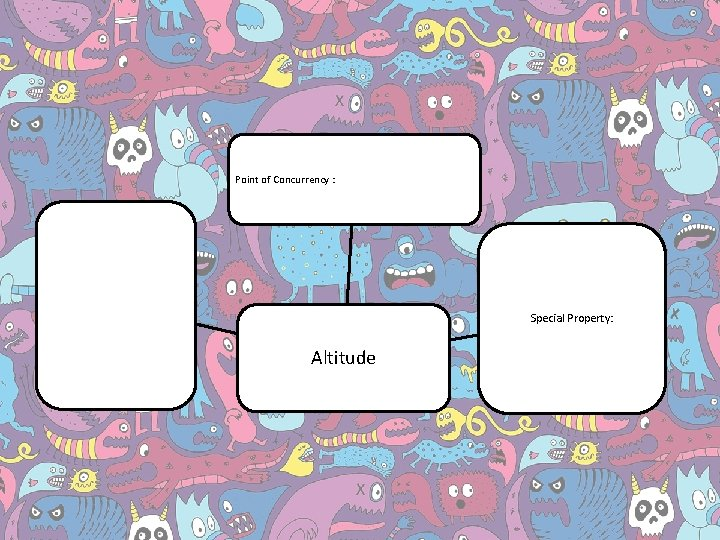Point of Concurrency : Special Property: AltitudeSummary! •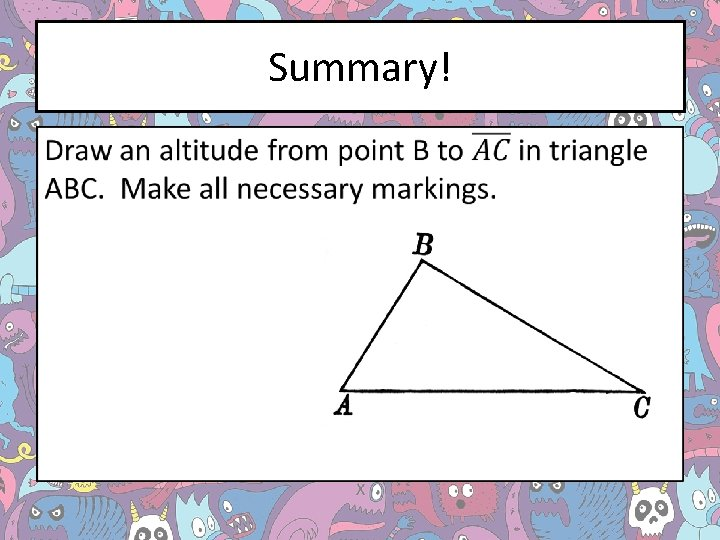Summary! •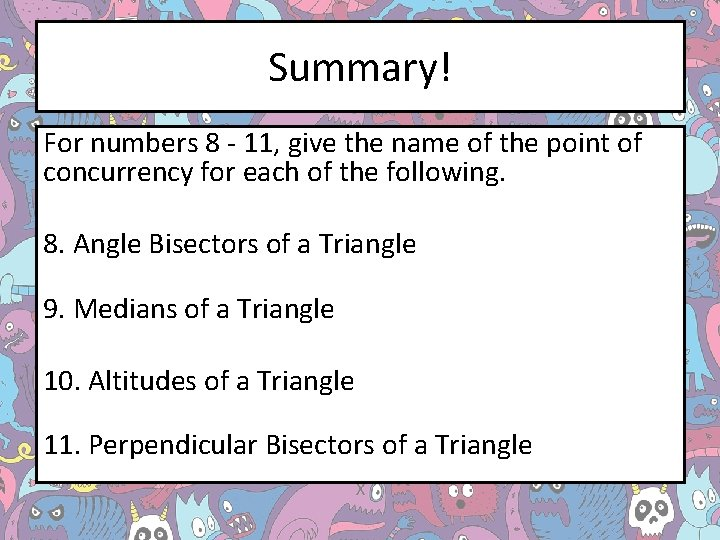Summary! For numbers 8 - 11, give the name of the point of concurrency for each of the following. 8. Angle Bisectors of a Triangle 9. Medians of a Triangle 10. Altitudes of a Triangle 11. Perpendicular Bisectors of a Triangle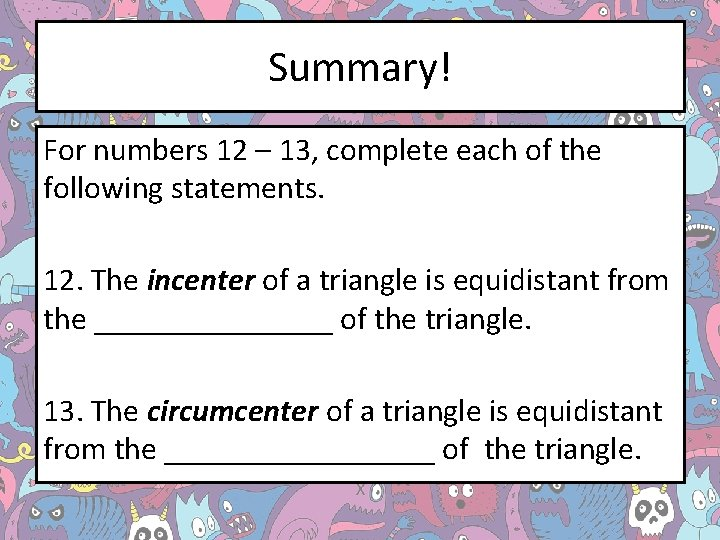Summary! For numbers 12 – 13, complete each of the following statements. 12. The incenter of a triangle is equidistant from the ________ of the triangle. 13. The circumcenter of a triangle is equidistant from the _________ of the triangle.